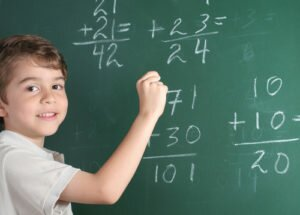# Multiplication Can Be Simple! – with a handout game!Multiplication is an operation that requires you to add another number to itself a certain number of times as indicated in the multiplication equation.

When students first start learning the concept of multiplication, it is more simple as time goes on for kids to learn. Memorizing multiplication facts works for some students but not for all! Some students need to learn by using different models and representations. When students have a conceptual understanding of multiplication and realize that it is connected to the real world, they tend to perform better on assessments. If a child is only ever taught isolated facts or memorized facts, they risk the chance of not understanding the meaning behind the objects they are multiplying. Knowing a variety of ways to solve multiplication problems will allow a student to figure out which strategy works best for them.

Math topics grow from one to another. If a child has mastered addition concepts then they can easily use repeated addition as a way to begin multiplying simple numbers. Repeated addition works to solve multiplication problems by adding the same number many times. Rather than forcing a child to memorize multiplication facts, they work by adding simple numbers using models to help arrive at the total or product for the problem.

To practice using this method the student will simply add a given number a certain number of times as indicated in the equation. For example, when multiplying 3 x 4, the student will recognize that the first addend stands for the number of objects in a group and the second addend represents how many times you will add. So, to solve the multiplication equation of 3 x 4, you will add 3+3+3+3. There are 3 objects in each group added together four times. The sum of 3+3+3+3 is 12 so 3 x 4 = 12.

Practice pages include instructions on each page.

Multiplication-Handout (WORD .doc)